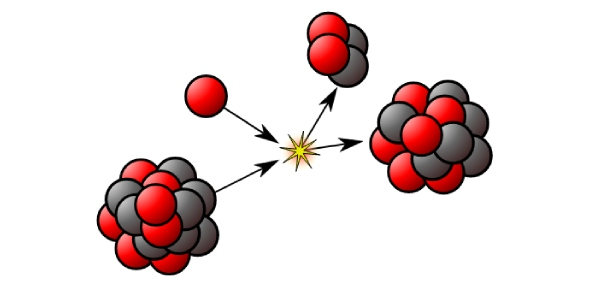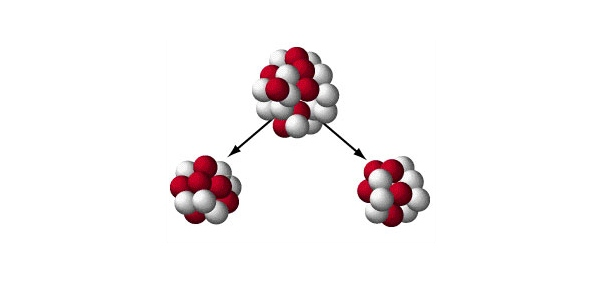How can you define the term nuclear ratio? - ProProfs Discuss+ Ask Question

# How can you define the term nuclear ratio?

Asked by M. Jabrowsky, Last updated: Nov 09, 2020

###Request 0FollowShareAnswer AnonymouslyAnswer LaterCopy LinkJ. Rogers, Rosshazlewood, MA, BirminghamThe nuclear ratio of an atomic nucleus is equal to the ratio of the number of the atomic neutrons to the number of the atomic protons. This can also be referred to as the neutron-proton ratio or N/P ratio. Considering the stable nuclei, the higher their atomic number, the higher the amount of this nuclear ratio.

This is due to the fact that the forces of electrical repulsive between protons scale are stronger than the nuclear force attractions. Precisely, most pairs of protons that are present in large nuclei are not distanced from themselves enough, and the electrical repulsion dominates over those strong nuclei forces.

Therefore, in stable larger nuclei, the proton density will be lower than that of stable smaller nuclei, where which more pairs of protons have short-ranged attractions of nuclear force. Taking oxygen, for example, in relation to our nuclear ratio definition; the number of neutrons and protons of oxygen are both 8. Then the nuclear ratio would be 8/8, which is equal to 1.#### Samantha Stewart

Love to do some charity work. Have a passion for writing and do it in my spare timeSamantha Stewart, Philanthropist, Post Graduate, Corpus Christi

The nuclear ratio, or neutron-proton ratio, of an atomic nucleus is the ratio of its number of neutrons to its number of protons. Among stable nuclei and naturally occurring nuclei, this ratio builds with increasing atomic number. This happens because electrical repulsive forces between protons scale with distance differently than strong nuclear force attractions.Most pairs of protons particularly in large nuclei are not far enough apart, such that electrical repulsion rules over the strong nuclear force. Hence, proton density in stable larger nuclei must be lower than in stable smaller nuclei where more pairs of protons have appreciable short-range nuclear force attractions.For each element with atomic number Z small enough to occupy only the first three nuclear shells, that is up to that of calcium (Z = 20), there exists a stable isotope with N/Z ratio of one, with the exception of beryllium (N/Z = 1.25) and every element with odd atomic number between 9 and 19 inclusive (N = Z+1). Hydrogen-1 (N/Z ratio = 0) and helium-3 (N/Z ratio = 0.5) are the only stable isotopes with neutron–proton ratio under one. Uranium-238 and plutonium-244 have the highest N/Z ratios of any primordial nuclide at 1.587 and 1.596, respectively, while lead-208 has the highest N/Z ratio of any known stable isotope at 1.537.Search for Google imagesSelect a recommended image
Upload from your computerCancelSearch for Google imagesSelect a recommended image
Upload from your computerCancelSearch for Google imagesSelect a recommended image
Upload from your computerCancel